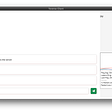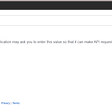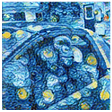# Introduction

What happens in most companies, is you start of with a GREAT spread of customers over your sales force. Then, over time, the waters get muddied and a few years down the line you probably have something similar to figure 1. Note the different colors, denotes different rep’s and see how much they overlap. This article will deal with sorting this mess out.

If you have not worked with KMEAN’s, then here is a good article.

# Required packages

We will be using KMEAN’s as our initial algorithm to group the customers according to their locations. However, usual KMEAN’s doesn’t quite do the trick in our scenario, since KMEAN’s can end up with very unbalanced area’s. One rep could end up with 20 customers and another could end up with 150. So k-means-constrained to the rescue. We can give minimum and maximum for each area. So, first up, install using pip.

`pip install k-means-constrained`

Now, lets import the packages we need

`import numpy as npimport pandas as pdimport matplotlib.pyplot as pltimport foliumfrom k_means_constrained import KMeansConstrained`

Now import your data into a data frame as I have below in figure 2. I have blocked out some data, but you get the point, you need the following fields:

• AccountID — the customers account numer
• RepID — The sales representative linked to the customer
• Latitude
• Longitude
• Location — A simple concat of latitude,longitude

# Plotting the initial data

You can use below to plot the existing customer base with each representative as a different colour.

`lst_reps = sorted(list(dfm.RepID.unique()))k = len(lst_reps) - 1# Get our list of unique clusterslst_elements = sorted(list(dfm.RepID.unique()))# Now make up random colors for each unique RepIDlst_colors = ['#%06X' % np.random.randint(0, 0xFFFFFF) for i in range(len(lst_elements))]# Add color column to dataframe and apply colorsdfm["color"] = dfm["RepID"]dfm["color"] = dfm["color"].apply(lambda x:lst_colors[lst_elements.index(x)])m = folium.Map(tiles="cartodbpositron", zoom_start=11)for index, row in dfm2.iterrows():  folium.CircleMarker(    location=[float(row['Latitude']),        float(row['Longitude'])],radius=4,popup=str(row['AccountID']+'|'+str(row['RepID'])+'|'+row['Name']+'|'+row['UserField01']),    color=row['color'],    fill=True,    fill_color=row['color'],).add_to(m)m`

# Training parameters

Let’s set up out training parameters.

• K indicates the number of representatives
• We want a minimum of 45 customers per representative
• A maximum of 92 customers per representative
`lst_reps = sorted(list(dfm.RepID.unique()))k = len(lst_reps) -2min_customers = 45max_customers = 92`

# KMEAN’s to cluster our customers

Now, lets set up our KMEAN’s parameters and run fit_predict. Importantly, we will want to save the centroids for each cluster.

In our case, each centroid will be a central location for each representatives area or territory.

`## Create a new dataframe with latitude/longitudeX = dfm[['Latitude','Longitude']]df_X = X.copy()### KMeansConstrained parametersclf = KMeansConstrained(  n_clusters=k,  size_min=min_customers,  size_max=max_customers,  random_state=0)clf.fit_predict(df_X)# save resultsth_centroids = clf.cluster_centers_df_clustercentroids = pd.DataFrame(th_centroids)df_clustercentroids.columns = ['Latitude','Longitude']print(clf.inertia_)# send back into dataframe and display itdfm['cluster1'] = clf.labels_dfm.head()`

Your result is now as below, take note of the new fields in our dataframe

• cluster1 — The cluster

# Assign each cluster to the most common representative

A little like the highest common denominator, lets use an APPLY to find the most common representative in a cluster and assign them to the whole cluster.

`# get a copy of the dataframedfn = dfm.copy()clusters = dfn.cluster1.unique()# Remove unwanted repsdfn = dfn.loc[dfn['RepID'] != 2195]dfn = dfn.loc[dfn['RepID'] != -1]# Iterate through the unique clustersfor cluster in clusters:  # find the most common rep in the cluster  repid = dfn.loc[dfn.cluster1 == cluster].RepID.mode() #.loc  if repid.size == 1:    repid = int(repid.loc)  else:    repid = 9999  # assign the cluster to its repid  print(str(repid) + ':' + str(cluster))  dfm.loc[dfm.cluster1 == cluster,'RepID1'] = repid  # Remove rep from dataframe copy so that its not used again  dfn = dfn.loc[dfn.RepID != repid]dfm = dfm.astype({"RepID1": int})`

You can also see a count of how many customers have been clustered per representative:

`dfm.groupby(['RepID1','cluster1'])['AccountID'].count()`

# Update centroids

Let’s update the repID’s to the centroid as well, just for info purposes

`df_clustercentroids['cluster'] = df_clustercentroids.indexdf_clustercentroids['RepID'] = df_clustercentroids.apply(lambda row: -1 if dfm.loc[dfm.cluster1==row.cluster].RepID1.mode().size == 0 else dfm.loc[dfm.cluster1==row.cluster].RepID1.mode(),axis=1)df_clustercentroids.head(20)`

# Plot the results

Now, lets plot the results and see how we are fairing

`# Get our list of unique clusterslst_elements = sorted(list(dfm.cluster1.unique()))# Now make up random colors for each unique clusterlst_colors = ['#%06X' % np.random.randint(0, 0xFFFFFF) for i inrange(len(lst_elements))]  # Add color column to dataframe and apply colors  dfm["color"] = dfm["cluster1"]  dfm["color"] = dfm["color"].apply(lambda x:  lst_colors[lst_elements.index(x)])m = folium.Map(tiles="cartodbpositron", zoom_start=11)for index, row in dfm.iterrows():  folium.CircleMarker(  location=[float(row['Latitude']), float(row['Longitude'])],  radius=4,  popup=str(row['cluster1']),  color=row['color'],  fill=True,  fill_color=row['color'],  tooltip=str(row['cluster1']) + '|' + row.AccountID).add_to(m)# Plot centroidsfor index, row in df_clustercentroids.iterrows():  folium.Marker(    location=row,    popup=str(index),    tooltip=str(index) + '|#=' +    str(dfm.loc[dfm.cluster1==index].groupby(['cluster1']    ['AccountID'].count().iloc)).add_to(m)m`

As you can see, we are already doing much better and plotting the centroids also gives us the central location for each area.

# So, whats the problem?

Well, KMEANS-constrained is great, but the constraints now cause a few outliers which we can use KNN to resolve. Figure 5 highlights the issue for you. Can you see one customer assigned to the brown representative, BUT it doesn’t make sense…. It should actually be with the purple representative.

Easy to spot on a map and lets use KNN to resolve this. If you do not know the KNN algorithm, then have a look at this article.

# KNN to the rescue

First up, lets setup a train and test dataset.

`# Import train_test_split functionfrom sklearn.model_selection import train_test_split# Split dataset into training set and test setX_train, X_test, y_train, y_test = train_test_split(dfm[['Latitude','Longitude']], dfm[['cluster1']], test_size=0.3) # 70% training and 30% test`

Now that we classified our data using KMEAN’s, lets train a KNN model to predict based on the KMEAN’s results. This will help us with the following

1. Identify the outliers from KMEAN’s due to its min/max constraints.
2. Have a model we can use going forward to predict which representative an account should be assigned to.

Now that we trained, lets run a prediction on our test set and then measure accuracy. We should see above 92% accuracy of our model

`#Import knearest neighbors Classifier modelfrom sklearn.neighbors import KNeighborsClassifierfrom sklearn import metrics#Create KNN Classifierknn = KNeighborsClassifier(n_neighbors=k)#Train the model using the training setsknn.fit(X_train, y_train)#Predict the response for test datasety_pred = knn.predict(X_test)# Model Accuracy, how often is the classifier correct?print("Accuracy:",metrics.accuracy_score(y_test, y_pred))`

# Predict on ALL data

We have a KNN model, so lets predict for latitude/longitude on our entire dataset. This will help show us some outliers where KMEAN’s made an odd choice due to the constraint of min/max customers per rep.

`y_pred = knn.predict(dfm[['Latitude','Longitude']])`

Update the predictions to our dataframe and mark the failed knnfailer=true when KNN did not agree with KMEANS. New clusters will be in the cluster2 column.

`dfm['cluster2'] = y_preddfm['outlier'] = dfm.apply(lambda row: False if row.cluster1 == row.cluster2 else True, axis=1)dfm[dfm.cluster1 != dfm.cluster2]`

Note the results in figure 6 with our 2 new columns. The handy outlier field tells us if KNN changed the customer to a new area.

# Update RepID2 from most common RepID1 in cluster

In this step, lets create a repid2 in the cluster are all assigned to the most common RepID1 in the cluster 1. This ensures the outliers get the correct rep code into RepID2.

`def getMostCommonRep(row):  global dfm  repid = dfm.loc[dfm.cluster1 == row.cluster2].RepID1.mode()  if repid.size == 1:    return repid.loc  else:    return -1dfm['RepID2'] = dfm.apply(lambda row: getMostCommonRep(row), axis=1)dfm = dfm.astype({"RepID": 'int32'})dfm = dfm.astype({"RepID1": 'int32'})dfm = dfm.astype({"RepID2": 'int32'})dfm = dfm.astype({"cluster1": 'int32'})dfm = dfm.astype({"cluster2": 'int32'})dfm.head(20)`

# Lets plot the new results

Now lets plot our results to see if KNN solved our outlier problem:

`# Get our list of unique clusterslst_elements = sorted(list(dfm.cluster2.unique()))# Now make up random colors for each unique clusterlst_colors = ['#%06X' % np.random.randint(0, 0xFFFFFF) for i in  range(len(lst_elements))]# Add color column to dataframe and apply colorsdfm["color"] = dfm["cluster2"]dfm["color"] = dfm["color"].apply(lambda x:lst_colors[lst_elements.index(x)])m = folium.Map(tiles="cartodbpositron", zoom_start=11)for index, row in dfm[dfm.outlier == False].iterrows():  folium.CircleMarker(location=[float(row['Latitude']), float(row['Longitude'])],  radius=4,  popup=str(row['cluster2']),  color=row['color'],  fill=True,  fill_color=row['color'],  tooltip=str(row['cluster2']) + '|' + str(row['RepID2']) + '|' + row.AccountID  ).add_to(m)# Plot centroidsfor index, row in dfm[dfm.outlier == True].iterrows():  folium.CircleMarker(location=[float(row['Latitude']), float(row['Longitude'])],  radius=7,  popup=str(row['cluster2']),  color='black',  fill=True,  fill_color='black',  tooltip= 'old=' + str(row['cluster1']) + '| new=' + str(row['cluster2']) + '|' + row.AccountID).add_to(m)m`

Great, as you can see in figure 7, our outlier has been reassigned to the purple representative. Note, we do give it a black border so we can easily pick the outliers out.

# Conclusion

I hope this article will help you on using machine learning to cluster your customers geographically. As with most machine learning, you may need more than just one algorithm to get thee right result.

# Sources

A big thanks to this article that got me onto kmeans-constrained. It was a big help.

--

--

--

## More from Geek Culture

A new tech publication by Start it up (https://medium.com/swlh).

## The Top 5 Books to Learn Java## Dissecting Networking in the Chat Demo app built with Teverse## A Simple GIS Server## Coding for FinTech## DevOps as an enabler for digital sales## Using REST APIs with Dasha.ai## Fall on Battlefield Servers: How to Solve Connection Problems in Battlefield 2042## Shaun Enslin

Coding, technology, data, crypto & lots of cycling are my passions. https://twitter.com/Shaunenslin https://www.linkedin.com/in/shaun-enslin-4984bb14b/

## Why you need Data Science to do Day Trading: A Bovespa example.## ML approach for Bitcoin swing trading — Part 3— Classification with Trend Labelling## Using Python, Data Science and Classic Time Series models to create a trading algorithm for the US…## Time Series Stock Prediction with LSTM